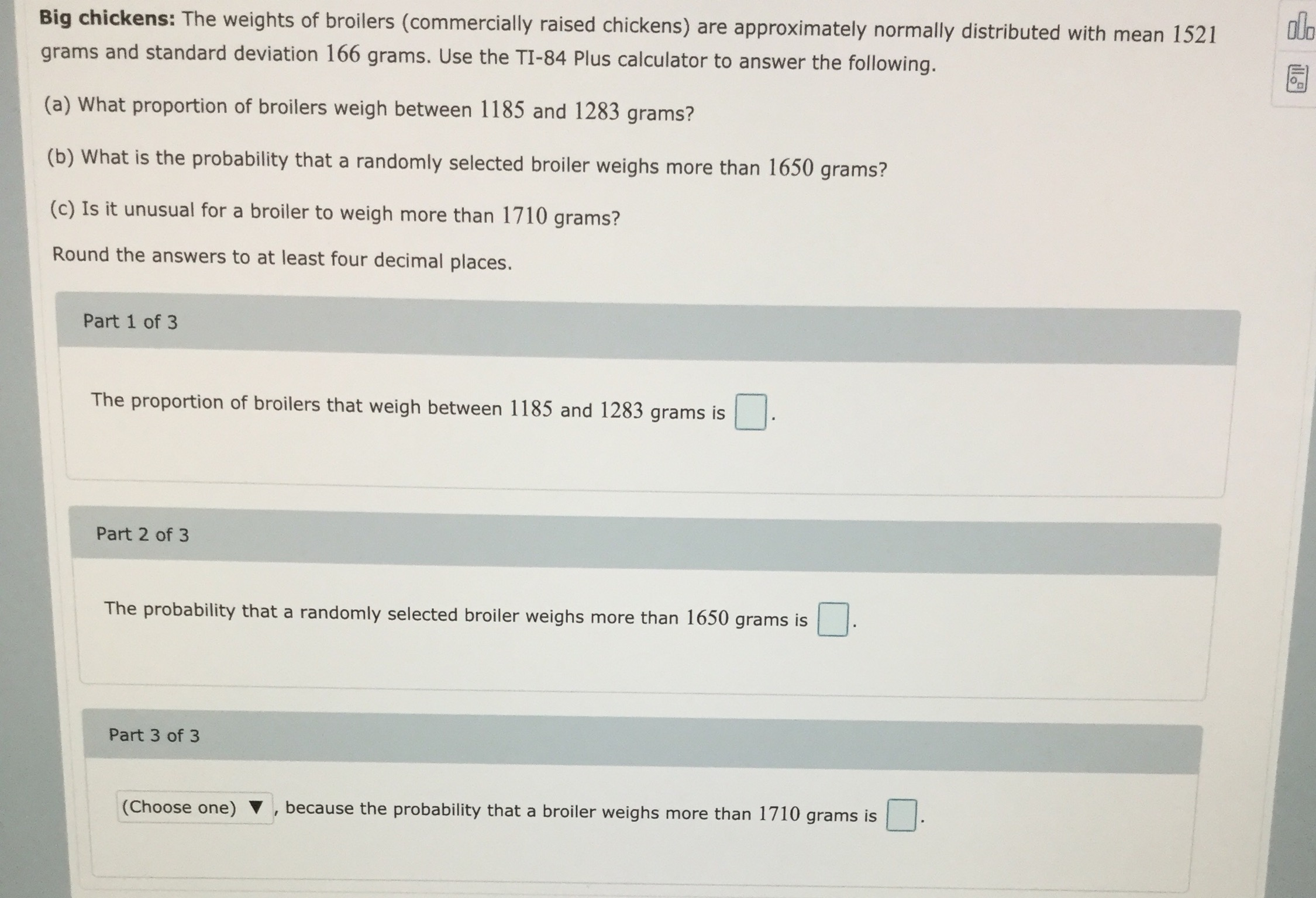# Big chickens: The weights of broilers (commercially raised chickens) are approximately normally distributed with mean 1521 dlo grams and standard deviation 166 grams. Use the TI-84 Plus calculator to answer the following. (a) What proportion of broilers weigh between 1185 and 1283 grams? (b) What is the probability that a randomly selected broiler weighs more than 1650 grams? (c) Is it unusual for a broiler to weigh more than 1710 grams? Round the answers to at least four decimal places. Part 1 of 3 The proportion of broilers that weigh between 1185 and 1283 grams is Part 2 of 3 The probability that a randomly selected broiler weighs more than 1650 grams is Part 3 of 3 because the probability that a broiler weighs more than 1710 grams is (Choose one) V

Questionhelp_outlineImage TranscriptioncloseBig chickens: The weights of broilers (commercially raised chickens) are approximately normally distributed with mean 1521 dlo grams and standard deviation 166 grams. Use the TI-84 Plus calculator to answer the following. (a) What proportion of broilers weigh between 1185 and 1283 grams? (b) What is the probability that a randomly selected broiler weighs more than 1650 grams? (c) Is it unusual for a broiler to weigh more than 1710 grams? Round the answers to at least four decimal places. Part 1 of 3 The proportion of broilers that weigh between 1185 and 1283 grams is Part 2 of 3 The probability that a randomly selected broiler weighs more than 1650 grams is Part 3 of 3 because the probability that a broiler weighs more than 1710 grams is (Choose one) V fullscreen

## Expert Answer

### Want to see this answer and more?

Experts are waiting 24/7 to provide step-by-step solutions in as fast as 30 minutes!*

*Response times may vary by subject and question complexity. Median response time is 34 minutes for paid subscribers and may be longer for promotional offers.
Tagged in
Math
Statistics© 2021 bartleby. All Rights Reserved.